Get our FREE VBA eBook of the 30 most useful Excel VBA macros.

Automate Excel so that you can save time and stop doing the jobs a trained monkey could do.

# AGGREGATE: The best Excel function you’re not using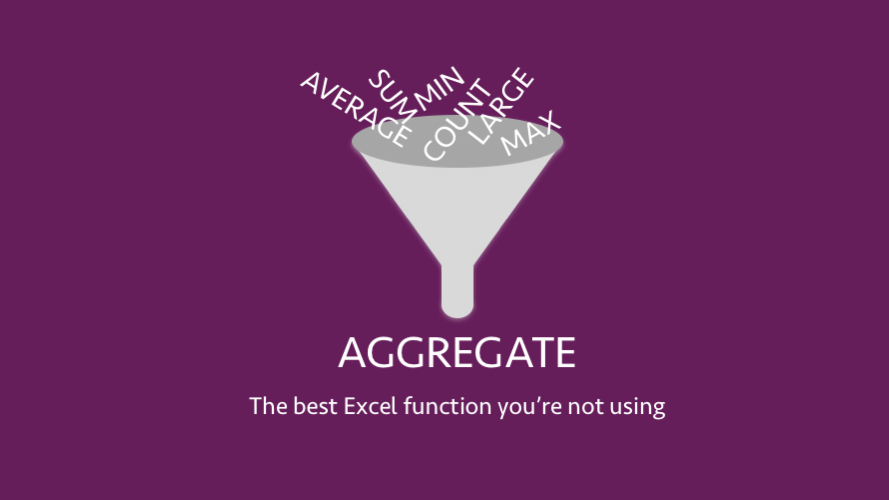In Excel 2010, Microsoft introduced a new function called AGGREGATE. It is one of the most powerful functions in Excel, yet I’ve rarely seen it used in a real-life scenario.  My aim with this post is to show you how good AGGREGATE is, so that you can make use of its power.

## What does AGGREGATE do?

Its name is deceptive. What does the word aggregate mean? To form a whole from separate parts.  That sounds the same as SUM doesn’t it?  Hence the problem with its name, the AGGREGATE function does so much more than SUM.

AGGREGATE can COUNT, AVERAGE, MAX, SMALL and SUM, to name just a few.  But it’s better than those functions because it performs all of those whilst ignoring errors, hidden cells and other cells containing AGGREGATE  and SUBTOTAL functions.

To help you see the power, let’s consider some scenarios.

### Scenario 1

Let’s say you want to find the 2nd largest result over a range of cells where those cells contain an #N/A error.  We would typically turn to the LARGE function for this type of calculation, but in this circumstance, LARGE will return an error.

We can tell the AGGREGATE function to ignore the error,  enabling it to calculate the correct result.

The screenshot below shows the result of both the LARGE and AGGREGATE functions.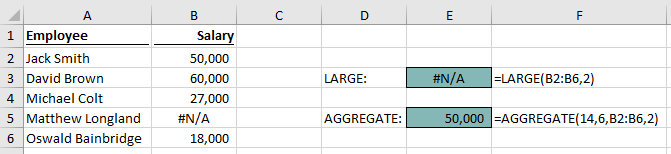### Scenario 2

As a second example, what if you want to find the average of the visible cells.  The AVERAGE function will calculate using all the cells in the range; it does not care whether those cells are visible or not.

We can tell the AGGREGATE function to ignore hidden cells, enabling it to calculate the correct result.

The screenshot below, in which row 5 is hidden, shows the average of the visible cells should be 38,750 (as calculated by AGGREGATE), not 71,000 (as calculated by AVERAGE).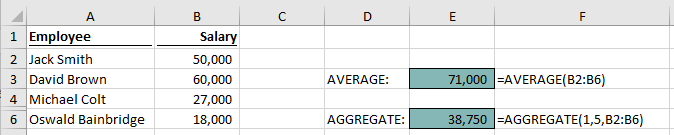### Bonus feature

As a bonus, AGGREGATE can also process arrays for specific functions without the need for Ctrl + Shift + Enter. If you don’t know what that means, don’t worry. But if you do, you’ll know this is a big deal.

## How to use AGGREGATE

The AGGREGATE function can have two syntax forms:

Reference form:

`=AGGREGATE(function_num,options,ref1,[ref2],...)`

Array form:

`=AGGREGATE(function_num,options,array,[k])`

You do not need to worry about which form you are using.  Excel will adopt the necessary form based on the function_num selected.

Let’s consider each of the arguments in the function.

### function_num

A value from 1 to 19 which indicates the type of action to be performed.

 function_num Calculation performed Description Function Form 1 AVERAGE Returns the average (mean) Reference 2 COUNT Counts number of values or cells which contain numbers Reference 3 COUNTA Counts number of values or cells which are not empty Reference 4 MAX Returns the largest value Reference 5 MIN Returns the smallest value Reference 6 PRODUCT Multiplies all the numbers Reference 7 STDEV.S Estimates standard deviation based on a sample Reference 8 STDEV.P Calculates standard deviation based on a population Reference 9 SUM Adds all the values or cells in a range Reference 10 VAR.S Estimates the variance based on a sample Reference 11 VAR.P Calculates variance based on a population Reference 12 MEDIAN Returns the median (the number in the middle) Reference 13 MODE.SNGL Returns the most frequently occurring number Reference 14 LARGE Returns the nth largest value Array 15 SMALL Returns the nth smallest value Array 16 PERCENTILE.INC Returns the nth percentile of values in a range which includes the value Array 17 QUARTILE.INC Returns the quartile based on a percentile including the value Array 18 PERCENTILE.EXC Returns the nth percentile of values in a range which excludes the value Array 19 QUARTILE.EXC Returns the quartile based on a percentile excluding the value Array

### options

A value from 0 to 7 indicating the cells to include within the function

 options Description 0 or [blank] Ignore nested SUBTOTAL and AGGREGATE functions 1 Ignore hidden rows, nested SUBTOTAL and AGGREGATE functions 2 Ignore error values, nested SUBTOTAL and AGGREGATE functions 3 Ignore hidden rows, error values, nested SUBTOTAL and AGGREGATE functions 4 Ignore nothing 5 Ignore hidden rows 6 Ignore error values 7 Ignore hidden rows and error values

### ref1 /  [ref2]

ref1: a reference to the range of cells on which the function is to be applied

[ref2]: an optional additional range of cells. There can be up to 252 separate ranges (including ref1), each separated by a comma ( , ).

### array

An array of values or an array formula upon which the function is to be applied

### [k]

A selection criteria which applies to specific function_nums

 calculation Description LARGE The nth largest value to be found SMALL The nth smallest value to be found PERCENTILE.INC The percentage value, must be between 0 and 1 QUARTILE.INC The quartile, must be 0, 1, 2, 3, 4 PERCENTILE.EXC The percentage value, must be between 0 and 1 QUARTILE.EXC The percentage, must be 0, 1, 2, 3, 4

## Too many things to remember?

If you think that AGGREGATE is complicated because there are too many things to remember, don’t worry.   When you start typing the function into a cell or in the formula box, a drop-down will appear with the various options.

The function_num drop-down list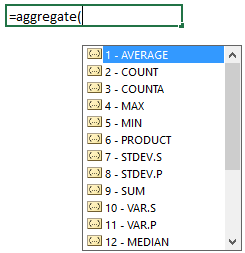The options drop-down list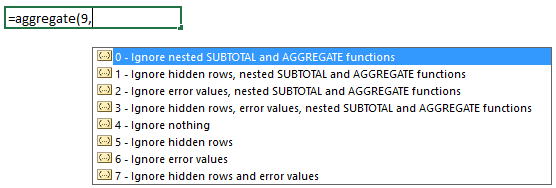If you decide to apply the function through the fx button, it is much less helpful.It requires you to select the format of the of the function (though if you select the wrong form and apply the arguments in the right positions, it will still calculate correctly).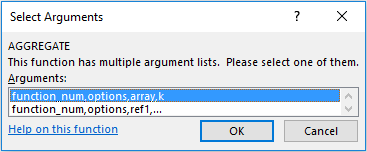The Function Arguments Window does not provide any guidance on the function_num or options.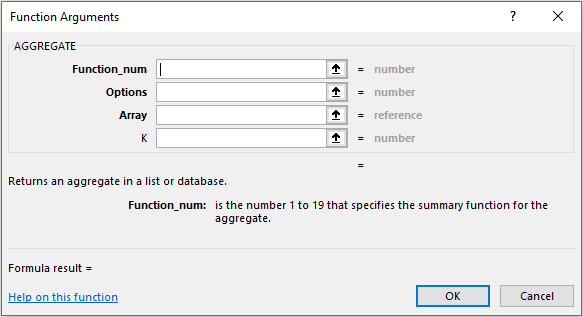Therefore, to use the AGGREGATE function, it is best to type directly into the cell or formula box, rather than using the fx button.## Examples

Now that you understand the function a bit, we can start to look at a few examples.Notice that row 4 is hidden and Cell D6 contains an error.

### Example 1 – Multiple references

The formula in Cell B12 is:

`=AGGREGATE(1,7,C2:C9,D2:D9,E2:E9)`

This formula is calculating the average (function_num = 1) of cells C2-C9, D2-D9 and E2-E9 whilst ignoring hidden rows and errors (options = 7).

Whilst it is possible to include a single reference of C2:E9, this example shows each column of values individually.

### Example 2 – LARGE

The formula in Cell B14 is:

`=AGGREGATE(14,5,C2:C9,1)`

This formula is calculating the 1st largest (function_num = 14) value in the Range C2-C9 whilst ignoring hidden rows (options = 5).

### Example 3 – LARGE array formula avoiding Ctrl + Shift + Enter

This example is much more complex.  If you do not understand what it means, don’t worry.  You can still use AGGREGATE without understanding this example.

The formula in Cell B16 is:

`=AGGREGATE(14,5,C2:C9*(B2:B9="St Johns"),1)`

This example is an extension of Example 2 above.  It now includes the requirement that 1st largest only be calculated over students from the St Johns school.

If we tried to achieve the same result with the standard LARGE function we would need to press Ctrl + Shift + Enter, because it is an array formula.  An example can be seen below, do not enter the curly braces, Excel will add those itself.

`{=LARGE(C2:C9*(B2:B9="St Johns"),1)}`

AGGREGATE does not need Ctrl + Shift + Enter to be used when using the function_num 14  to 19.

Before you get too excited, there is an issue here.  How is it possible that the largest value for St Johns is 72 when in Example 2 the largest value for all schools was 68?

This is a quirk of array formulas.  The 72 returned for St Johns is contained within the hidden row.  We selected option 5 to ignore hidden rows, but this setting has been ignored.

The problem is the calculation chain.  Excel will evaluate the formula in the following order:

1. B2:B9=”St Johns” = {TRUE;FALSE;TRUE;FALSE;TRUE;TRUE;FALSE;FALSE}
2. C2:C9*{TRUE;FALSE;TRUE;FALSE;TRUE;TRUE;FALSE;FALSE} = {55;0;72;0;58;60;0;0}
3. =AGGREGATE(14,5,{55;0;72;0;58;60;0;0},2)
4. =72

The array part of the function is calculated first, turning the cell references into values.  As a result, there are no cell references to ignore.  This is not necessarily a calculation error; we just need to understand how the formula works.

### Example 4 – Without ignoring errors

The formula in Cell B18 is:

`=AGGREGATE(9,5,D2:D9)`

This formula is trying to SUM (function_num = 9) the values, in Cells D2:D9 whilst ignoring hidden rows (options = 5).   The result of the formula is an #N/A error because the cells included also include an error.

## Common errors / problems

As with all functions, there are some common pitfalls and problem.

• If a [k] argument is required, but not provided a #VALUE error will appear. For example, when applying the LARGE function_num, there needs to be an argument to find the nth largest item.
• Whilst there is an option to ignore hidden rows, there is no option to ignore hidden columns.
• When using an array which involves a calculation, the ignore hidden rows option is ignored (as shown in Example 3 above).

## If it’s good, why not use AGGREGATE all the time?

If I have convinced you that AGGREGATE is a fantastic formula, then why not use it all the time?

There are a few drawbacks:

• It’s more complicated to use than it’s standard counterpart (i.e., SUM is easy to use, but to summing with AGGREGATE is a bit tricker)
• Takes longer to input all the arguments
• Too many options to remember
• Most users do not understand the function.
• Will not work for users with Excel 2007 or before

But there are just as many good reasons to use it too.

• Can do 152 different things (19 functions x 8 options), rather than just 1 (it’s a Swiss Army knife formula).
• Don’t need to fix formula errors in the range used within the function
• Calculations can be performed on the visible cells of filtered lists
• Can handle array formulas for specific function_num’s
• It’s a very fast formula

There you have it, that’s AGGREGATE, the best function you’re not using . . . until today.Get our FREE VBA eBook of the 30 most useful Excel VBA macros.

Automate Excel so that you can save time and stop doing the jobs a trained monkey could do.

Don’t forget:

If you’ve found this post useful, or if you have a better approach, then please leave a comment below.

Do you need help adapting this to your needs?

I’m guessing the examples in this post didn’t exactly meet your situation.  We all use Excel differently, so it’s impossible to write a post that will meet everybody’s needs.  By taking the time to understand the techniques and principles in this post (and elsewhere on this site) you should be able to adapt it to your needs.

But, if you’re still struggling you should:

1. Read other blogs, or watch YouTube videos on the same topic.  You will benefit much more by discovering your own solutions.
2. Ask the ‘Excel Ninja’ in your office.  It’s amazing what things other people know.
3. Ask a question in a forum like Mr Excel, or the Microsoft Answers Community.  Remember, the people on these forums are generally giving their time for free.  So take care to craft your question, make sure it’s clear and concise.  List all the things you’ve tried, and provide screenshots, code segments and example workbooks.
4. Use Excel Rescue, who are my consultancy partner.   They help by providing solutions to smaller Excel problems.

What next?
Don’t go yet, there is plenty more to learn on Excel Off The Grid.  Check out the latest posts:

## 12 thoughts on “AGGREGATE: The best Excel function you’re not using”

1.Will says:

Unreal, I can’t believe I’ve never heard of this. This will be super helpful. Thank you!

•Excel Off The Grid says:

Thanks Will.

That’s one convert… now you’ve just got to tell all of your work colleagues about it too.

2.Hareshkumar Shah says:

•Excel Off The Grid says:

I’m sorry it wasn’t working for you. I’ll send you a copy.

3.David says:

Excellent examples that really helped me understand AGGREGATE a bit more, but how would I use it to ignore zero values?

•Excel Off The Grid says:

AGGREGATE doesn’t have an option for ignoring zero values. Therefore you’ll need to think about other formula combinations to ignore zeros.

4.Tim says:

A valuable read. Thanks, I will add that one to the toolbox.

5.Svanius says:

Very cool function, thank you for sharing such detailed how to.

6.Kumari Kahatuduwa says:

I have never thought about “Aggregate” this way. Thanmks Mark.

7.Rahul Laxman Yadav says:

Good one. Liked the function thanks for sharing. It will help me in many ways.

8.Nix says:

I completely forgot about this function! I look forward to trialling it out in the future!

9.Brendon Carvill says:

I am still trying to see why I would use this over SUM. If an error exists I don’t want to ignore it.